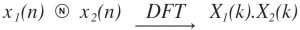View Course Path

# Properties of DFT (Summary and Proofs)

All of these properties of the discrete Fourier transform (DFT) are applicable for discrete-time signals that have a DFT. Meaning these properties of DFT apply to any generic signal x(n) for which an X(k) exists. (x(n)$\underleftrightarrow { \quad DFT\quad }$ X(k)) where$X(k)=\sum _{ n=0 }^{ N-1 }{ x(n){ e }^{ \frac { -j2\pi kn }{ N } } }$.

 Property Mathematical Representation Linearity a1x1(n)+a2x2(n)$\underleftrightarrow { \quad DFT\quad }$ a1X1(k) + a2X2(k) Periodicity if x(n+N) = x(n) for all n then x(k+N) = X(k) for all k Time reversal x(N-n)$\underleftrightarrow { \quad DFT\quad }$ X(N-k) Duality x(n)$\underleftrightarrow { \quad DFT\quad }$ Nx[((-k))N] Circular convolutionCircular correlation For x(n) and y(n), circular correlation rxy(l) is rxy(l)$\underleftrightarrow { \quad DFT\quad }$ Rxy(k) = X(k).Y*(k) Circular frequency shift x(n)e2πjln/N$\underleftrightarrow { \quad DFT\quad }$ X(k+l) x(n)e-2πjln/N$\underleftrightarrow { \quad DFT\quad }$ X(k-l) Circular time shift x((n-l))N$\underleftrightarrow { \quad DFT\quad }$ X(k)e-2πjlk/N or X(k)WklN where W is the twiddle factor. MultiplicationComplex conjugate x*(n)$\underleftrightarrow { \quad DFT\quad }$ X*(N-k) Symmetry For even sequences, X(k) =$\sum _{ n=0 }^{ N-1 }{ }$ x(n)Cos(2πnk/N) For odd sequences, X(k) =$\sum _{ n=0 }^{ N-1 }{ }$ x(n)Sin(2πnk/N) Parseval’s theorem$\sum _{ n=0 }^{ N-1 }{ }$ x(n).y*(n) =$\frac { 1 }{ N } \sum _{ k=0 }^{ N-1 }{ }$ X(k).Y*(k)

## Proofs of the properties of the discrete Fourier transform

Linearity

Statements: The DFT of the linear combination of two or more signals is the sum of the linear combination of DFT of individual signals.

Proof: We will be proving the property:

a1x1(n)+a2x2(n)$\underleftrightarrow { \quad DFT\quad }$ a1X1(k) + a2X2(k)

Periodicity

Statement: For a given DFT and IDFT pair, if the discreet sequence x(n) is periodic with a period N, then the N-point DFT of the sequence (i.e X(k)) is also periodic with the period of N samples.

Proof: We will be proving the property

if x(n+N) = x(n) for all n
then x(k+N) = X(k) for all k

According to the definition of DFT, we have,

X(k) =$\sum _{ n=0 }^{ N-1 }{ x(n){ { W }_{ N }^{ nk } } }$ where k = 0, 1, 2, … N-1.

To prove periodicity, put k = k+N

X(k+N) =$\sum _{ n=0 }^{ N-1 }{ x(n){ { W }_{ N }^{ n(k+N) } } }$

Rewriting,$\sum _{ n=0 }^{ N-1 }{ x(n){ { W }_{ N }^{ n(k) } }{ { W }_{ N }^{ n(N) } } }$

Let’s calculate the twiddle factor${ W }_{ N }^{ nN } = { e }^{ -j2\piN/N }$ = 1

Thus,

X(k+N) = X(k)

Time reversal

Statement: This property basically points to the circular folding of a sequence in a clockwise direction. When this is done, the DFT of the sequence will also get circularly folded.

Proof: We will be proving the property

x(N-n)$\underleftrightarrow { \quad DFT\quad }$ X(N-k)

x(N-n) =$\sum _{ p=0 }^{ N-1 }{ x(N-n){ e }^{ \frac { -j2\pi nk }{ N } } }$

Put N-n=p, that gives us n=N-p; substituting in the above equation we get,

DFT[x(N-n)] =$\sum _{ p=0 }^{ N-1 }{ x(p){ e }^{ \frac { -j2\pi (N-p)k }{ N } } }$

The lower limit will be the same since a DFT is periodic.

Duality

Statement: The DFT of a sequence can be used to find its finite duration sequence.

Proof:

Circular convolution

Statement: The multiplication of two DFT sequences is equivalent to the circular convolution of their sequences in the time domain. (Note that this is NOT the same as the convolution property.)

Proof:

Circular correlation

Statement: The circular cross-correlation of two sequences in the time domain is equivalent to the multiplication of DFT of one sequence with the complex conjugate DFT of the other sequence.

Proof:

Circular frequency shift

Statement: Multiplication of a sequence by the twiddle factor or the inverse twiddle factor is equivalent to the circular shift of the DFT in the time domain by ‘l’ samples.

Proof:

Circular time shift

Statement: Shifting the sequence in time domain by ‘l’ samples is equivalent to multiplying the sequence in frequency domain by the twiddle factor.

Proof:

Multiplication of two sequences

Statement: The multiplication of two sequences in the time domain is equivalent to their circular convolution in the frequency domain.

Proof:

Complex conjugate property

Statement: The DFT of a complex conjugate of any sequence is equal to the complex conjugate of the DFT of that sequence; with the sequence delayed by k samples in the frequency domain.

Proof:

Symmetry

Statement: The DFT of an even sequence is purely real and the DFT of an odd sequence is purely imaginary.

Proof: